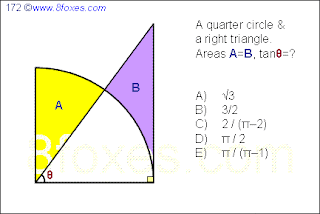## Saturday, October 31, 2009

### Fox 172

Simple beginnings...1.Notation: (S)=Area of S
theta=angle
(A)=(pi/2-theta)/2
(Right triangle) = tan(theta)/2
(C)=theta/2=(Right triangle) - (B)
(B)=tan(theta)/2-theta/2=(A)=(pi/2-theta)/2
tan(theta)-theta = pi/2 -theta -> tan(theta) = pi/2. -> Answer D.

MIGUE.

2.3.An easier way:
Call the white area C and the radius 1.
Call the hight of the right-angled triangle h.
Then because A(A)=A(B), is also A(A+C)=A(B+C).
This means that the area of the quarter circle is equal to the area of the right-angled triangle.
So: A(A+C)=pi/4.
A(B+C)=1*h/2 = pi/4 so h = pi/2.
tan(theta) = h/1 = h = pi/2 -> answer D.